# NCERT Solutions for Class 7 Maths Chapter 2 Fractions and Decimals Ex 2.5

NCERT Solutions for Class 7 Maths Chapter 2 Fractions and Decimals Ex 2.5

### NCERT Solutions for Class 7 Maths Chapter 2 Fractions and Decimals Ex 2.5

NCERT Solutions for Class 7 Maths Chapter 2 Fractions and Decimals Exercise 2.5
Ex 2.5 Class 7 Maths Question 1.
Which is greater?
(i) 0.5 or 0.05
(ii) 0.7 or 0.5
(iii) 7 or 0.7
(iv) 1.37 or 1.49
(v) 2.03 or 2.30
(vi) 0.8 or 0.88
Solution:
(i) 0.5 or 0.05
Comparing the tenths place, we get 5 > 0
∴ 0.5 > 0.05

(ii) 0.7 or 0.5
Comparing the tenths place, we get 7 > 5
∴ 0.7 > 0.5

(iii) 7 or 0.7
Comparing the one’s place, we get 7 > 0
∴ 7 > 0.7

(iv) 1.37 or 1.49
Comparing the tenths place, we get 3 < 4
∴ 1.37 < 1.49 or 1.49 > 1.37

(v) 2.03 or 2.30
Comparing the tenths place, we get 0 < 3
∴ 2.03 < 2.30 or 2.30 > 2.03

(vi) 0.8 or 0.88 ⇒ 0.80 or 0.88
Since tenths place is same.
Comparing the hundredth place, we get 0 < 8
∴ 0.80 < 0.88 or 0.88 > 0.80

Ex 2.5 Class 7 Maths Question 2.
Express as rupees using decimals:
(i) 7 paise
(ii) 7 rupees 7 paise
(iii) 77 rupees 77 paise
(iv) 50 paise
(v) 235 paise
Solution:Ex 2.5 Class 7 Maths Question 3.
(i) Express 5 cm in metre and kilometre
(ii) Express 35 mm in cm, m and km.
Solution:
(i) 1 metre = 100 cm
1 kilometre = 1000 metre = 100 × 1000 cm
= 100000 cm(ii) 1 cm = 10 mm and 1 km = 100000 cmEx 2.5 Class 7 Maths Question 4.
Express in kg:
(i) 200 g
(ii) 3470 g
(iii) 4 kg 8 g
Solution:Ex 2.5 Class 7 Maths Question 5.
Write the following decimal numbers in the expanded form:
(i) 20.03
(ii) 2.03
(iii) 200.03
(iv) 2.034
Solution:Ex 2.5 Class 7 Maths Question 6.
Write the place value of 2 in the following decimal numbers:
(i) 2.56
(ii) 21.37
(iii) 10.25
(iv) 9.42
(v) 63.352
Solution:Ex 2.5 Class 7 Maths Question 7.
Dinesh went from place A to place B and from there to place C. A is 7.5 km from B and B is 12.7 km from C. Ayub went from place A to place D and from there to place C. D is 9.3 km from A and C is 11.8 km from D. Who travelled more and by how much?
Solution:
Distance travelled by Dinesh from A to C
= AB + BC
= 7.5 km +- 12.7 km
= 20.2 kmDistance travelled by Ayub from A to C
= 9.3 km + 11.8 km = 21.1 km
Since 21.1 km > 20.2 km.
Hence, Ayub travelled more distance.

Ex 2.5 Class 7 Maths Question 8.
Shyama bought 5 kg 300 g apples and 3 kg 250 g mangoes. Sarala bought 4 kg 800 g oranges and 4 kg 150 g bananas. Who bought more fruits?
Solution:
Fruits bought by Shyama
= 5 kg 300 g apples + 3 kg 250 g mangoes
= 5.300 kg apples + 3.250 kg mangoes
= 8.550 kg of fruits
Fruits bought by Sarala
= 4 kg 800 g oranges +- 4 kg 150 g bananas
= 4.800 kg oranges + 4.150 kg bananas
= 8.950 kg of fruits
Since 8.950 kg > 8.550 kg
Hence, Sarala bought more fruits.

Ex 2.5 Class 7 Maths Question 9.
How much less is 28 km than 42.6 km?
Solution:
Since 28 km < 42.6 km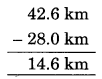Hence, 28 km is less than 42.6 km by 14.6 km.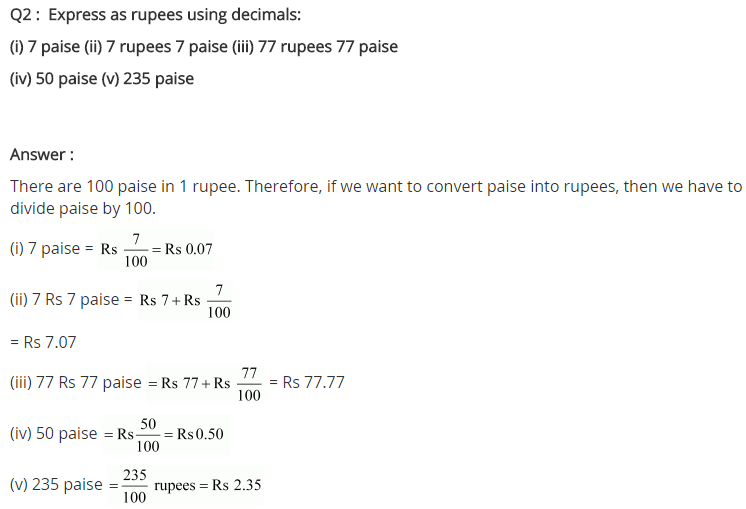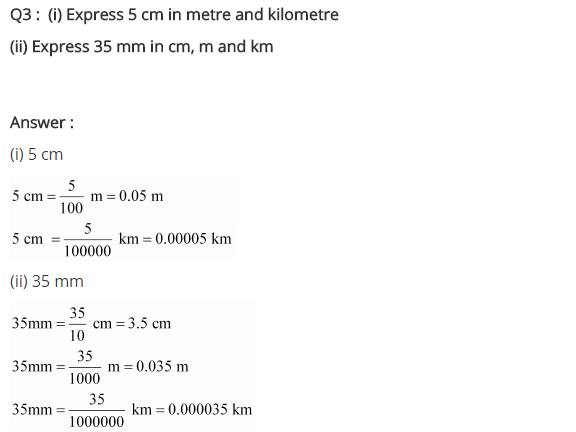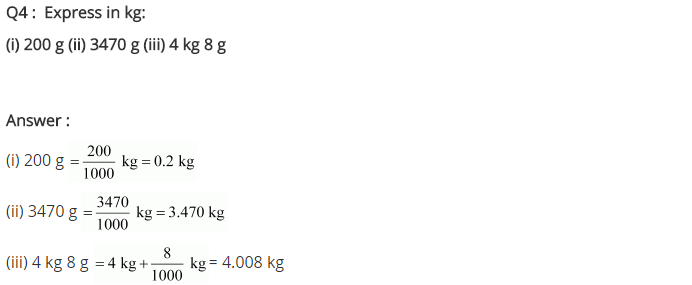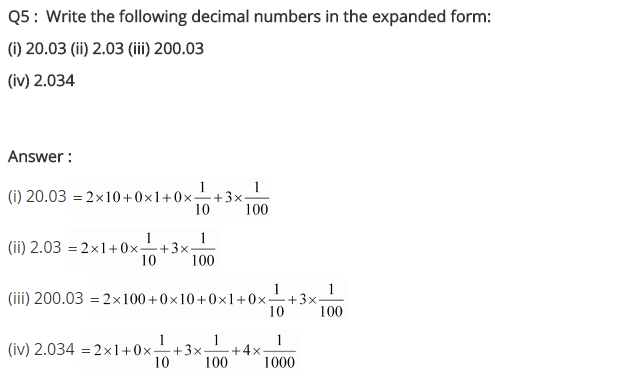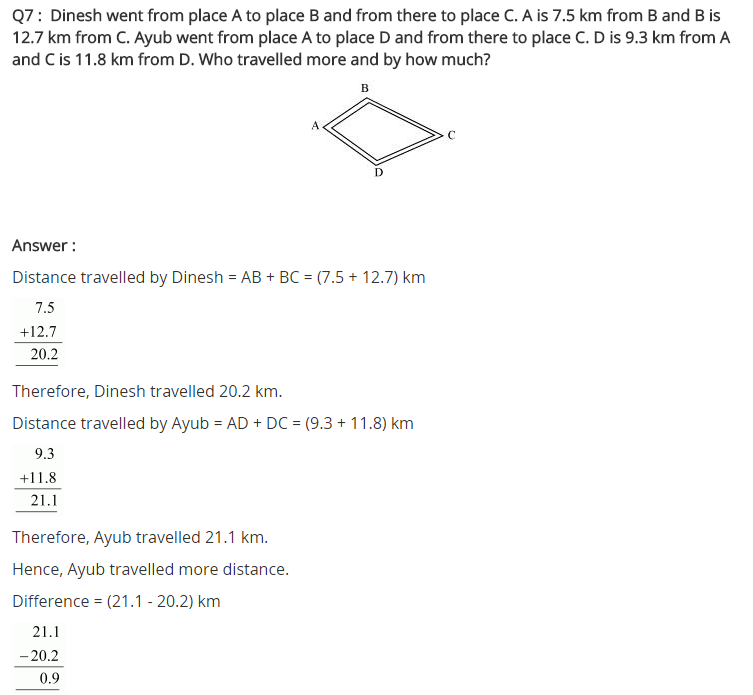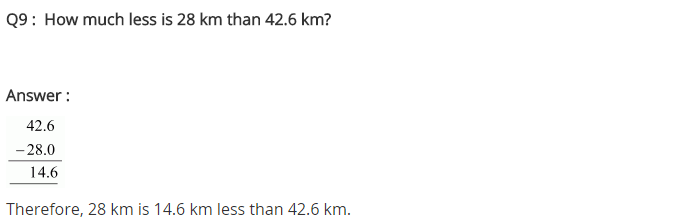## SabDekho

The Complete Educational Website